Updating search results...

# 9 Results

View
Selected filters:
• MCCRS.Math.Content.4.NBT.A.3
Remix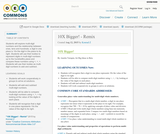Conditional Remix & Share Permitted
CC BY-NC-SA
Rating
0.0 stars

Students will explore multi-digit numbers and the relationship between ones, tens and hundreds; a digit in one place is 10x the digit in the place to its right. Students will use their bodies to represent digits in multi-digit numbers up to the hundredths place and compare these numbers using <, =, >. Students will use their bodies as multi-digit numbers to add and subtract.

Subject:
Mathematics
Material Type:
Activity/Lab
Teaching/Learning Strategy
Author:
08/08/2019
Remix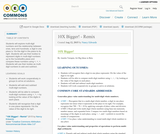Conditional Remix & Share Permitted
CC BY-NC-SA
Rating
0.0 stars

Students will explore multi-digit numbers and the relationship between ones, tens and hundreds; a digit in one place is 10x the digit in the place to its right. Students will use their bodies to represent digits in multi-digit numbers up to the hundredths place and compare these numbers using <, =, >. Students will use their bodies as multi-digit numbers to add and subtract.

Subject:
Mathematics
Material Type:
Activity/Lab
Teaching/Learning Strategy
Author:
Nancy Edwards
08/08/2019
Remix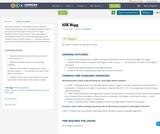Conditional Remix & Share Permitted
CC BY-NC-SA
Rating
0.0 stars

Students will explore multi-digit numbers and the relationship between ones, tens and hundreds; a digit in one place is 10x the digit in the place to its right. Students will use their bodies to represent digits in multi-digit numbers up to the hundredths place and compare these numbers using <, =, >. Students will use their bodies as multi-digit numbers to add and subtract.

Subject:
Mathematics
Material Type:
Activity/Lab
Teaching/Learning Strategy
Author:
Jody Bauer
08/08/2019
Remix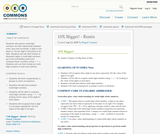Conditional Remix & Share Permitted
CC BY-SA
Rating
0.0 stars

Students will explore multi-digit numbers and the relationship between ones, tens and hundreds; a digit in one place is 10x the digit in the place to its right. Students will use their bodies to represent digits in multi-digit numbers up to the hundredths place and compare these numbers using <, =, >. Students will use their bodies as multi-digit numbers to add and subtract.

Subject:
Mathematics
Material Type:
Activity/Lab
Teaching/Learning Strategy
Author:
Raquel Vazquez
08/08/2019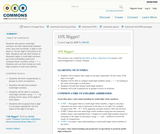Conditional Remix & Share Permitted
CC BY-NC-SA
Rating
3.5 stars

Students will explore multi-digit numbers and the relationship between ones, tens and hundreds; a digit in one place is 10x the digit in the place to its right. Students will use their bodies to represent digits in multi-digit numbers up to the hundredths place and compare these numbers using <, =, >. Students will use their bodies as multi-digit numbers to add and subtract.

Subject:
Mathematics
Material Type:
Activity/Lab
Teaching/Learning Strategy
Author:
01/28/2016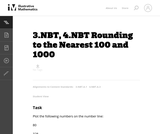Unrestricted Use
CC BY
Rating
0.0 stars

This is a task from the Illustrative Mathematics website that is one part of a complete illustration of the standard to which it is aligned. Each task has at least one solution and some commentary that addresses important asects of the task and its potential use. Here are the first few lines of the commentary for this task: Plot the following numbers on the number line: 80 328 791 1. Round each number to the nearest 100. How can you see this on the number line? 2. Round ea...

Subject:
Mathematics
Material Type:
Activity/Lab
Provider:
Illustrative Mathematics
Provider Set:
Illustrative Mathematics
Author:
Illustrative Mathematics
02/18/2014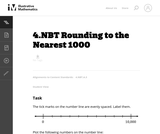Unrestricted Use
CC BY
Rating
0.0 stars

This is a task from the Illustrative Mathematics website that is one part of a complete illustration of the standard to which it is aligned. Each task has at least one solution and some commentary that addresses important asects of the task and its potential use. Here are the first few lines of the commentary for this task: The tick marks on the number line are evenly spaced. Label them. Plot the following numbers on the number line: 85 940 2,316 5,090 7,784 Round each num...

Subject:
Mathematics
Material Type:
Activity/Lab
Provider:
Illustrative Mathematics
Provider Set:
Illustrative Mathematics
Author:
Illustrative Mathematics
02/18/2014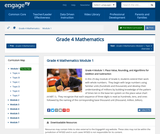Conditional Remix & Share Permitted
CC BY-NC-SA
Rating
0.0 stars

In this 25-day module of Grade 4, students extend their work with whole numbers.  They begin with large numbers using familiar units (hundreds and thousands) and develop their understanding of millions by building knowledge of the pattern of times ten in the base ten system on the place value chart (4.NBT.1).  They recognize that each sequence of three digits is read as hundreds, tens, and ones followed by the naming of the corresponding base thousand unit (thousand, million, billion).

Subject:
Numbers and Operations
Material Type:
Module
Provider:
New York State Education Department
Provider Set:
EngageNY
05/11/2013
Remix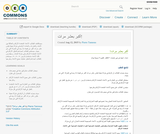Unrestricted Use
CC BY
Rating
0.0 stars

سيستكشف الطلاب الأعداد المتعددة الأرقام والعلاقة بين الآحاد والعشرات والمئات؛ الرقم في منزلة معينة يكون عشر مرات أكبر من قيمته إذا وجد في المنزلة التي عن يمينه. سيستخدم الطلاب أجسادهم لتمثيل الأرقام في الأعداد المتعددة الأرقام حتى منزلة المئات.
وسيقارنون تلك الأعداد باستخدام الرموز < ، = ، >. سيستخدم الطلاب أجسادهم لتمثيل عمليات جمع الأعداد وطرحها.

Subject:
Mathematics
Material Type:
Activity/Lab
Teaching/Learning Strategy
Author:
Pierre Tannous